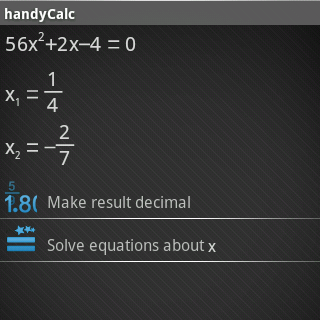# HandyCalc App for Google Android

June 23, 2009

Name: HandyCalc App for Google Android

Summary:

HandyCalc is a utility application in Android devices that is very powerful. It has the ability to solve mathematical and equation problems. It comes in almost all features that a usual calculator can have. Most of these features are still yet to explore. This app is very easy to use and learn as well.

HandyCalc is a utility application in Android devices that is very powerful. It has the ability to solve mathematical and equation problems

User Interaction:

Most of the mobile devices today have an installed calculator that performs the basic operations such as multiplication, division, addition, and subtraction. Some mobile devices are even installed with calculator that contains complicated or too many buttons. This kind of calculator is more known as the scientific calculator.

However, with HandyCalc app, every calculating function and complication is redefined making it easier for users to use. In addition, more complicated equations can be solved and comes with possible results. In any event that user left something behind, they are given the chance of finding them on the graphs.

User Interface:

Since the HandyCalc comes with more redefined interface compared to other calculator application, it becomes more convenient to use. Moreover, HandyCalc becomes more powerful and humanized to understand for its specific function. The tutorial of this application comes in three sections: he Basic Input and Calculation, the Advanced Mathematical Calculation, and the Useful Tricks.

Here is a list of features for HandyCalc application that you can use as interface guide:

• The app uses database in managing the pages instead of files.
• It uses the “Enter Key” in displaying the results directly.
• It uses system clipboard to copy and paste formulas into text format.
• It has keypad visibility for both portrait and landscape mode.
• It has GoTo and Zoom Mode function.
• It can support for non-decimal bases such as binary, octal, and hex.
• It uses multiplication symbol (x) on the G1 keyboard.
• It has updated demo function (help->demo).
• It has factory reset function in the preference menu.
•Utility and Productivity:

The HandyCalc app can be compared to GNU bc. It is useful for random calculations in programming since it supports all arithmetic operations that use 64-bit unsigned integers. It also performs conversions from binary to decimal to hex.

HandyCalc application is a result of intelligence and creativity. It is considered to be powerful because of the Android OS. HandyCalc is just similar to other calculators that perform simple arithmetic operations but it still considered to be unique because of its advanced mathematical features such as solving complex mathematical equations that include linear equations, finding the roots of an equation be it complex or linear, and performing calculations based on Newton methods.

With HandyCalc application, you can also draw, study, and compare graphs. The graphs can be animated without any problem. You just have to enter the equation where the graph is required and the application will manage the rest of the mathematical operations for you. HandyCalc will display the best possible graph available from the equation that you have entered. If you want to concentrate on a specific region of the graph, you can always zoom in and out from it.

Rating:

(9.0 out of 10) Despite the complex operations of HandyCalc application, it still keeps all complexities to itself. Its friendly and very clean interface makes it very easy to use even for novice users.

Category: Android Apps, Tools